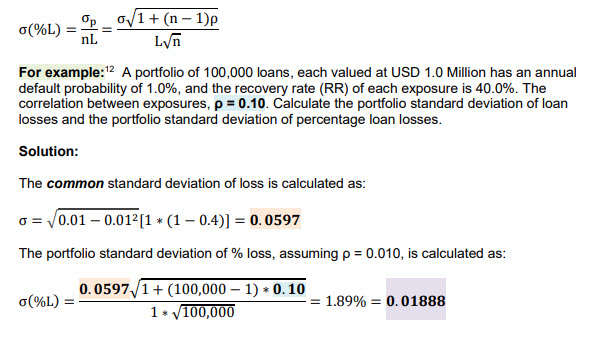# P1.T4 Ch 6 Credit Risk & Capital Modeling

#### etzaros

##### New Member
Hi David, I am struggling to understand some concepts of ch 6.
1. Please explain why in the formula of σp (portfolio standard deviation) you ignore the weight factor. It is known that the formula is (w1*σ1)^2+(w2*σ2)^2+2w1*w2*σ1*σ2*ρ. However, when you solve it you probably set w1=w2=w3=1, despite the fact that w1+w2+w3=1. I am referring to the example at page 25.
2. Please clarify me what is the correct formula for the standard deviaton of percentage loss, expressed as a percentage of the size of the portfolio. At page 16 you say it is σ(%L)=σ^2/(n*L). In the Garp book it is stated that the formula is σ(%L)=σ/(n*L). What is the correct approach?
3. Please clarify me something regarding copulas and Vasicek Model. At page 20 it is stated that Ui=α*F+sqrt(1-α^2)*Zi is the sum of two SND with μ=0 ανδ VAR= 1. However at page 88 at GARP book it is stated that the distribution of each Ui has μ=aF and σ=sqrt(1-a^2). My question is: at least Ui has a ND or a SND?

#### David Harper CFA FRM

##### David Harper CFA FRM
Staff member
Subscriber
Hi @etzaros

1. I think we just followed GARP's goofy example in 6.6 where implicitly the weights are \$1.0 rather than 33.3%. Notice their equation doesn't square any weights in referencing their 6.1 [sic] and only subsequently assumes all loans are the same size. So I think we just assumed all 1.0 to match their goofy approach. Yes you are correct that weights need to sum to 100%, but the dollar value of the position sizes can alternatively be used (which is tantamount to distributing the total portfolio over the weights).

2. The σ(%L) looks the same to me. I don't see your difference, sorry.3. The single-factor model U = α*F + sqrt(1 - α^2)*Z has a design intention the U be a SND. If F and Z are SND, ~N(0,1), and F and Z are uncorrelated, the variance(U) = variance(α*F) + variance[sqrt(1 - α^2)*Z] = α^2*variance(F) + (1 - α^2)*variance(Z) = α^2*1.0 + (1 - α^2)*1.0 = α^2 + (1 - α^2) = 1.0. This was tedious for me, cross-referencing GARP's materials, I will be slow to do that given my workload (you reference page numbers and i don't see the same things, not a good use of my time frankly), I hope it helped you,